# Hyper-graph Matching via Reweighted Random Walks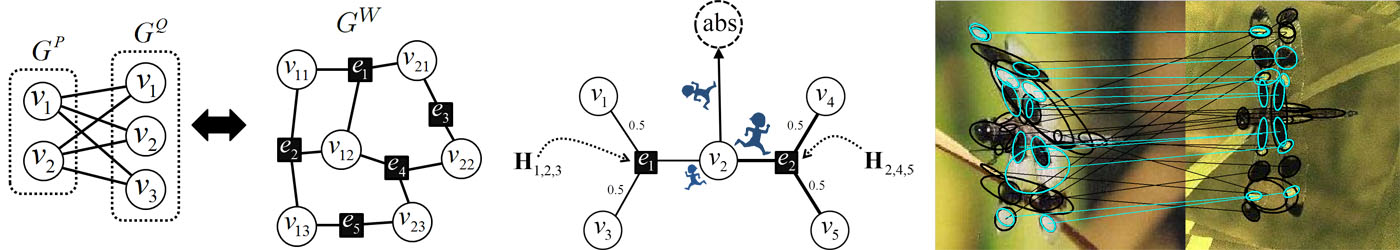## Abstract

Establishing correspondences between two feature sets is a fundamental issue in computer vision, pattern recognition, and machine learning. This problem can be well formulated as graph matching in which nodes represent feature points while edges describe pairwise relations between feature points. Recently, several researches have tried to embed higher-order relations of feature points by hyper-graph matching formulations. In this paper, we generalize the previous hyper-graph matching formulations to cover relations of features in arbitrary orders, and propose a novel state-of-the-art algorithm by reinterpreting the random walk concept on the hyper-graph in a probabilistic manner. Adopting personalized jumps with a reweighting scheme, the algorithm effectively reflects the one-to-one matching constraints during the random walk process. Comparative experiments on synthetic data and real images show that the proposed method clearly outperforms existing algorithms especially in the presence of noise and outliers.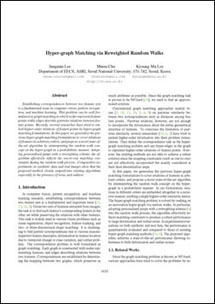## Paper

CVPR 2011 paper. (pdf, 5.51MB)

### Presentation

Poster (pdf), 438KB

### Code

zip file (MATLAB with C-mex) (zip), ver1.1 (2012.2.20), 725KB

### Datasets

Image dataset (zip), 10.2MB used in the real image matching of Sec.4.2

### Citation

Jungmin Lee, Minsu Cho, Kyoung Mu Lee. "Hyper-graph Matching via Reweighted Random Walks", Computer Vision and Pattern Recognition (CVPR), 2011.

### Previous Work

This work is an extension of RRWM which is provided in ECCV2010 here.

## Experimental Results

We evaluate the performances of several existing hyper-graph matching approaches by conducting experiments on both synthetic point-set data and real image data. For Hyper-Graph Matching (HGM) and Tensor Matching (TM) methods, we use the author provided MATLAB codes. We termed ours RRWHM, which is the abbreviation of Reweighted Random Walks Hyper-graph Matching. Our RRWHM is also implemented in MATLAB, and the parameters, α and β, are empirically tuned as 0.2 and 30, respectively. Each quantitative result in our synthetic experiments is acquired from averages of 100 random trials.

### Point-set Matching Test

In the point-set matching test, deformation noise and outliers are added to simulate a real matching problem. First, we randomly generate nin inlier points using the normal distribution N(0,1) in 2D domain P, which become nodes in the graph GP. To generate points in the second domain Q, the Gaussian deformation noise N(0,σ2) is independently added to the nin points. Outlier noise is simulated using additional nout random Gaussian points (i.e., N(0,1)) to each domain.
In the synthetic point-set matching experiment, it is impossible to directly compare two points by the unary similarity (δ = 1) since there is no distinctive descriptor for a single point. For pairwise cases (δ = 2), we can compare distances of two point sets to compute the pairwise similarity values. However, this measure is very sensitive to the scale change (i.e., invariant up to translation and rotation). To achieve scale invariance, we exploit triplets of correspondences (δ = 3) as described in Fig. 5 in the paper. In the entire experiment, we empirically set σs = 0.5 for the best performance.
When constructing the higher-order similarity tensor, the storage size of the tensor should be considered. Suppose we have m by m point-matching problem, then there will be m2 possible candidate correspondences. For the δ = 3 case, the size of the resulting full-affinity tensor will be (m23), an order of O(m6), which demands a huge amount of memory. Thus, in this work, to make the higher-order similarity tensor sparse, we sample n=nin+nout triangles per point and find n2 nearest neighbors using the approximate nearest neighbor searching algorithm.

 Deformation Test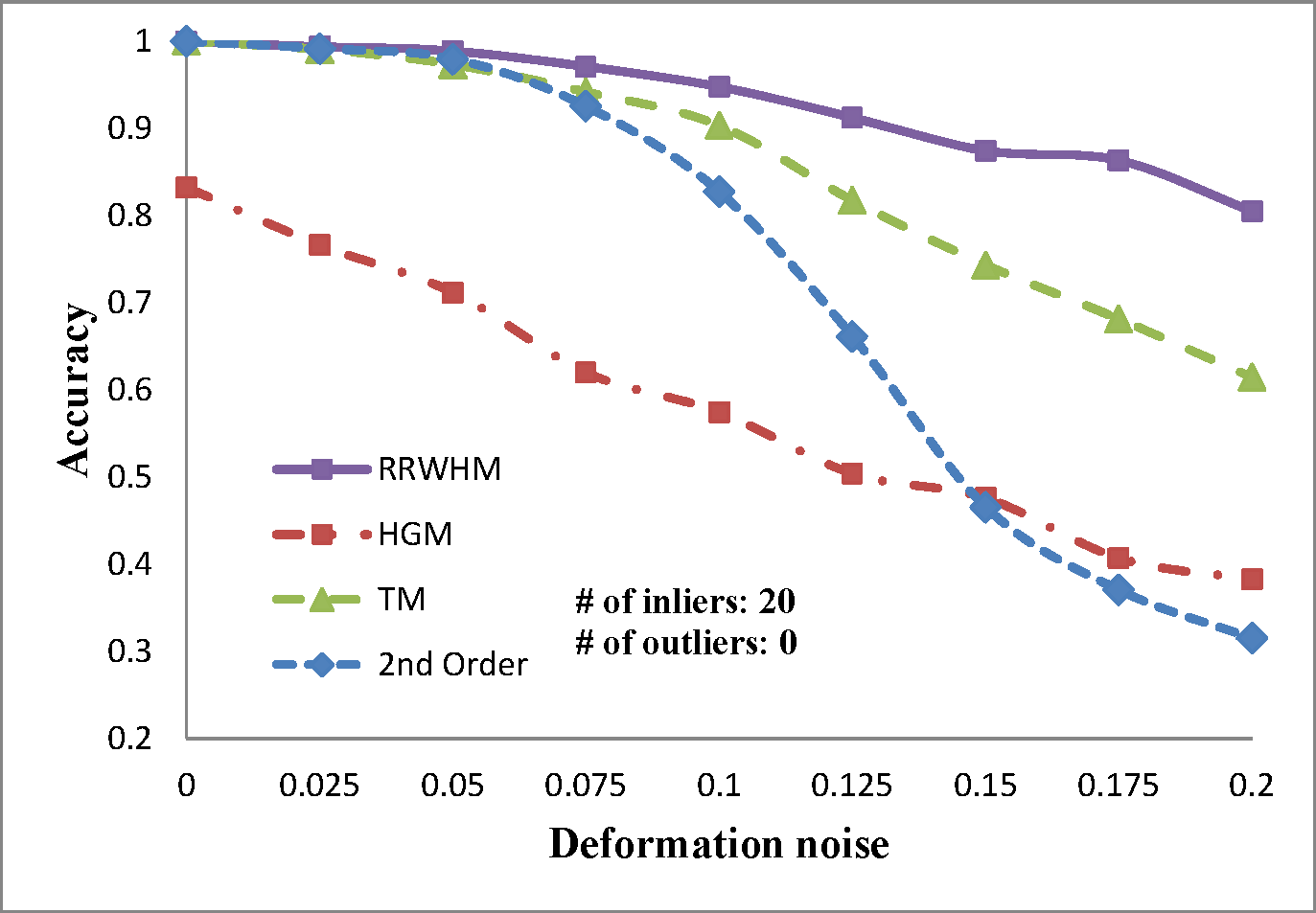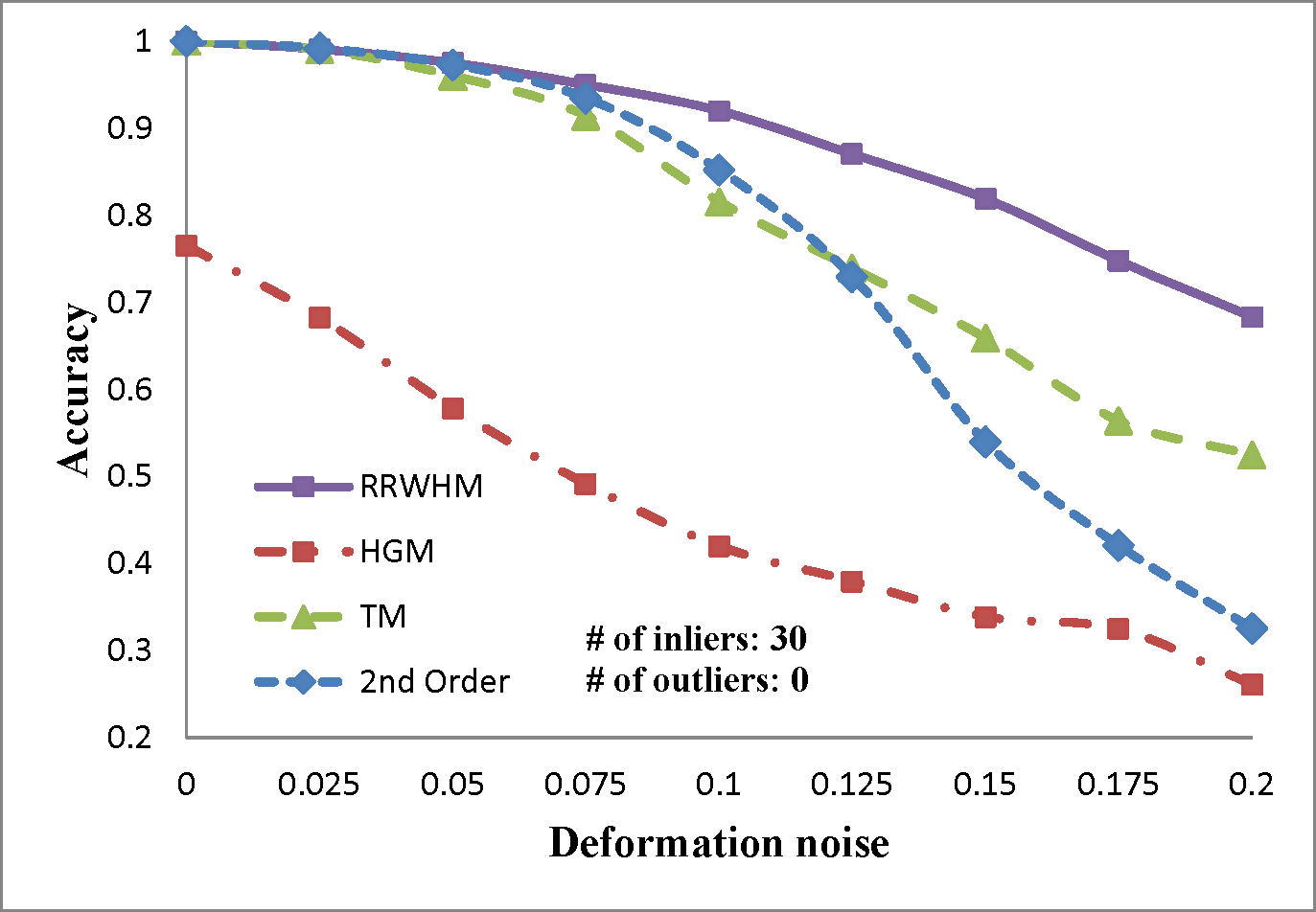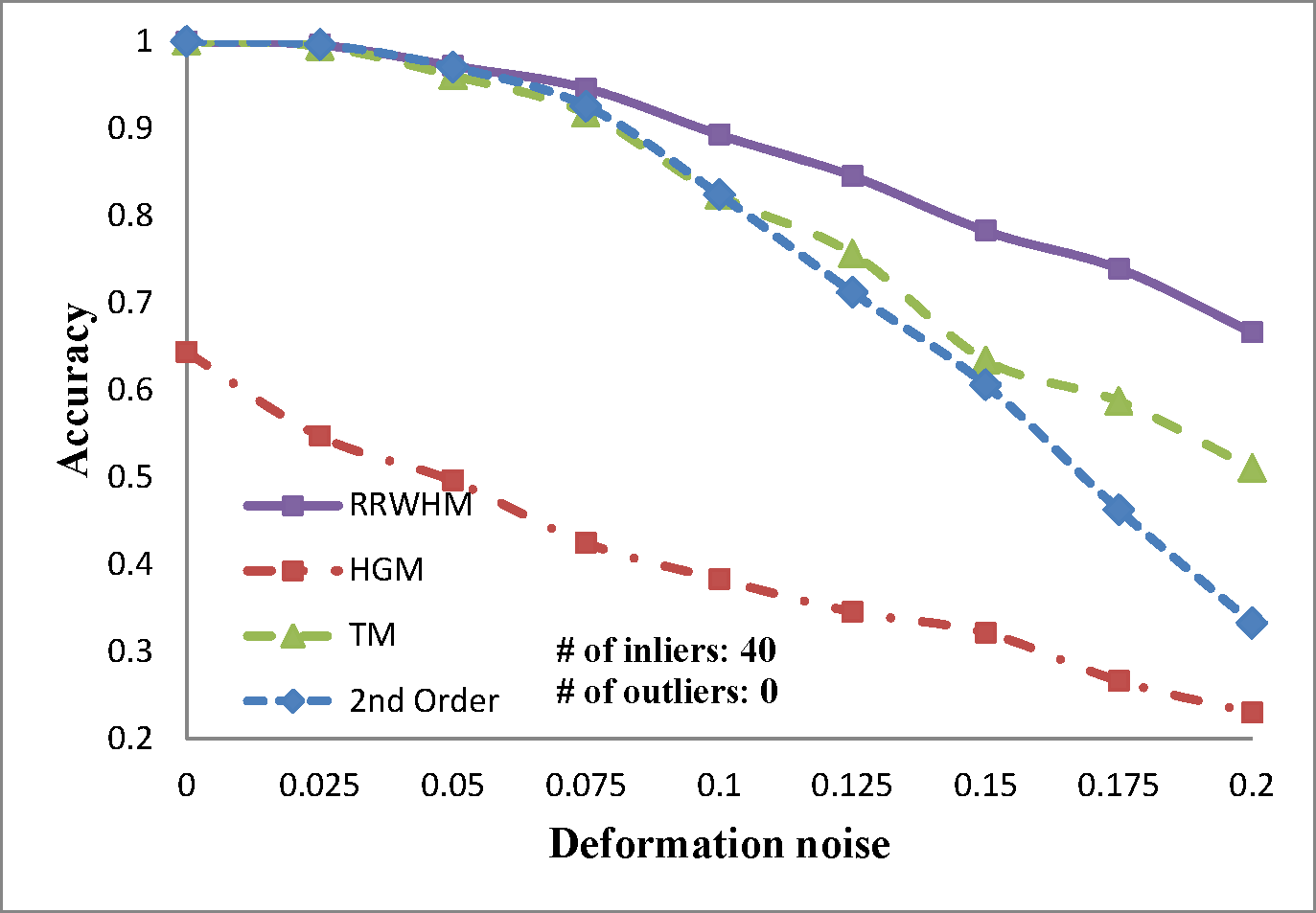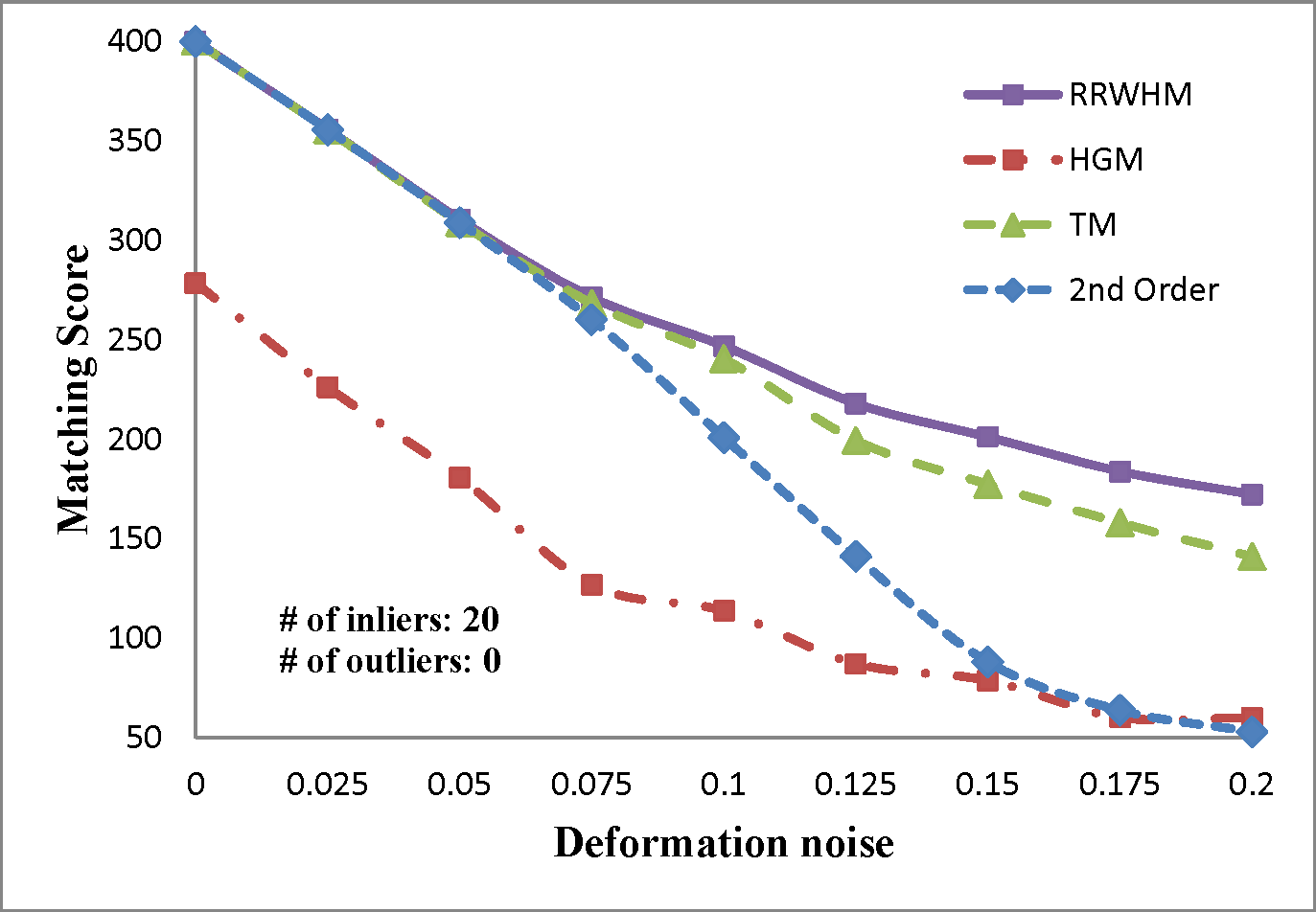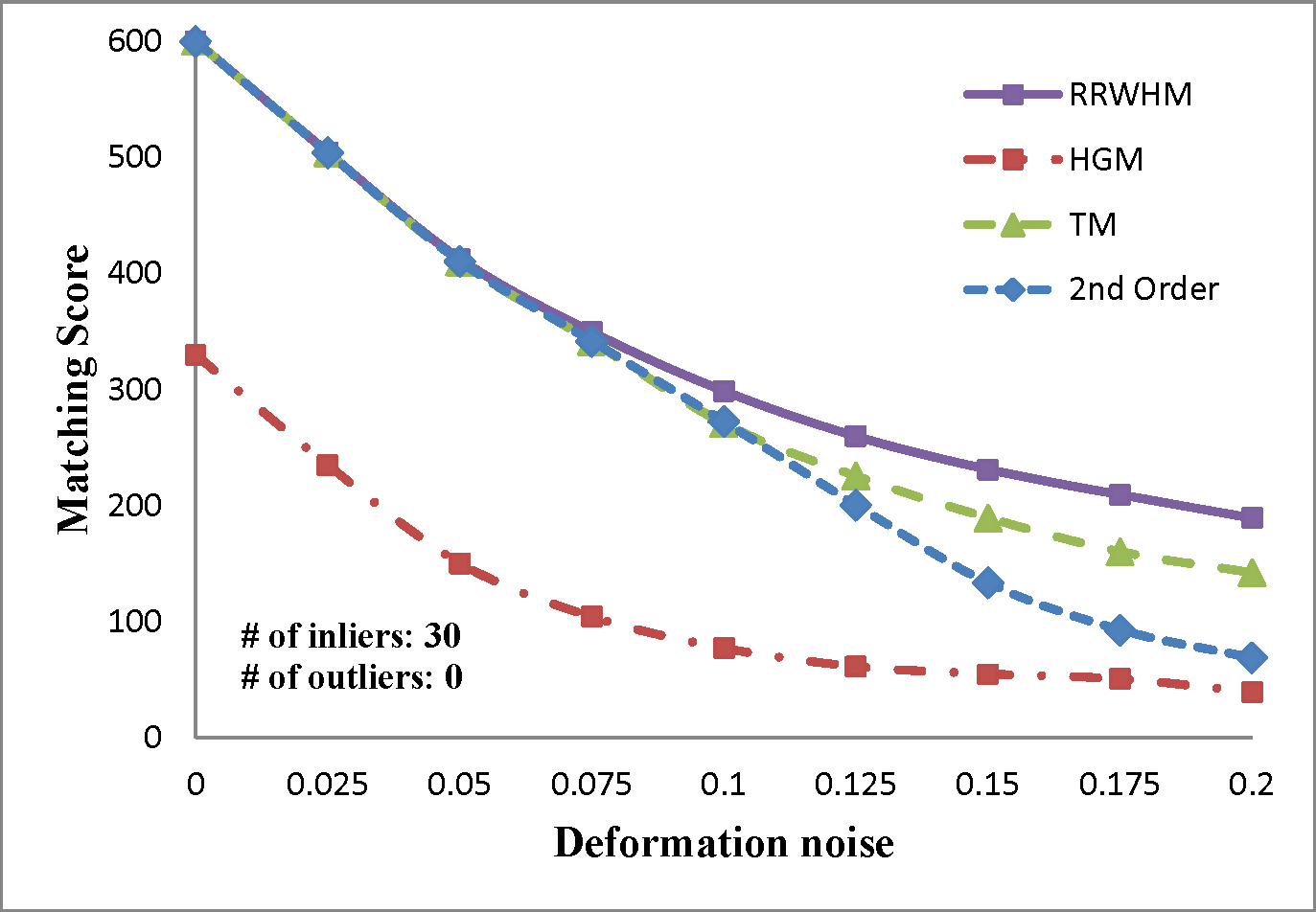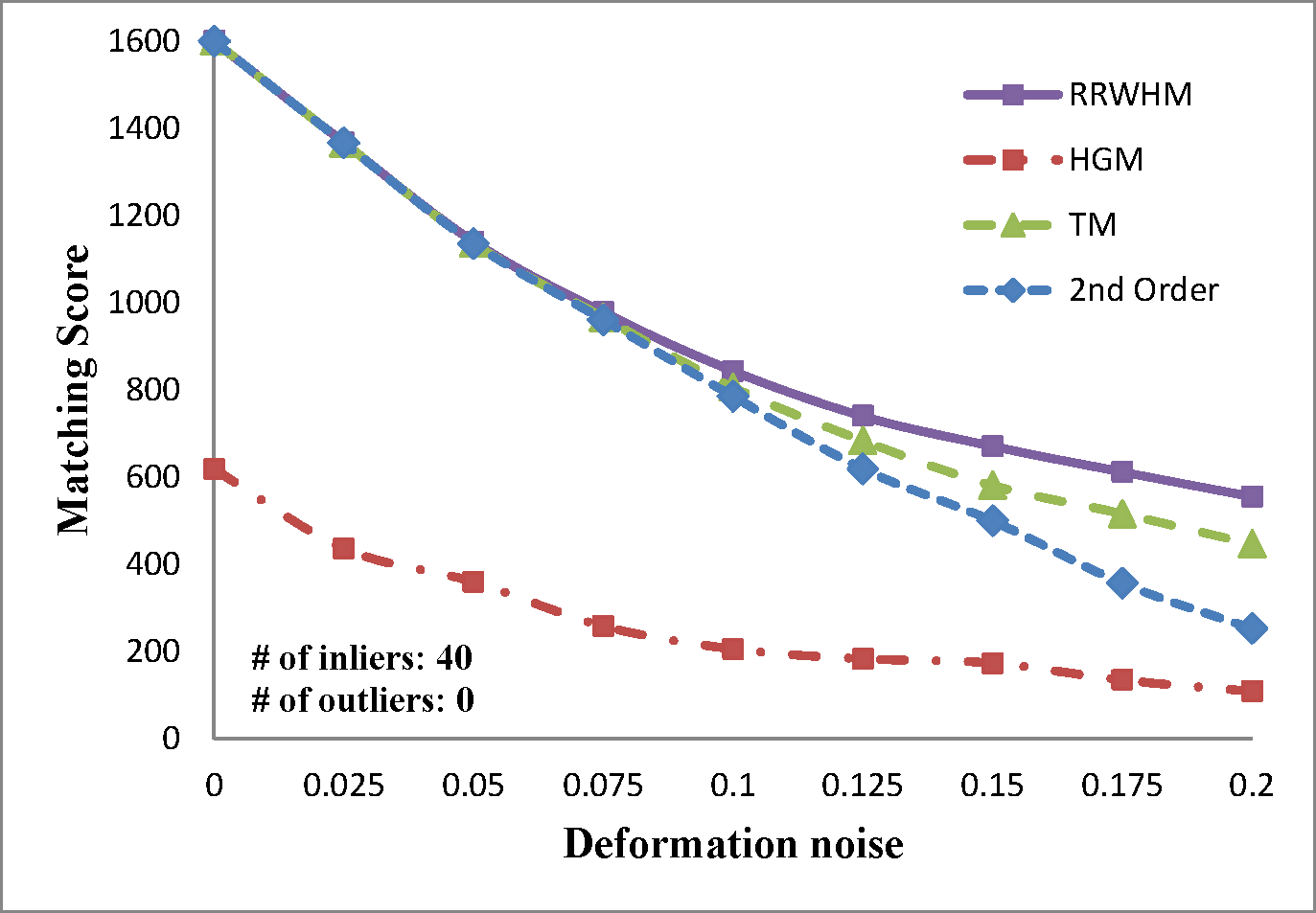Outlier Test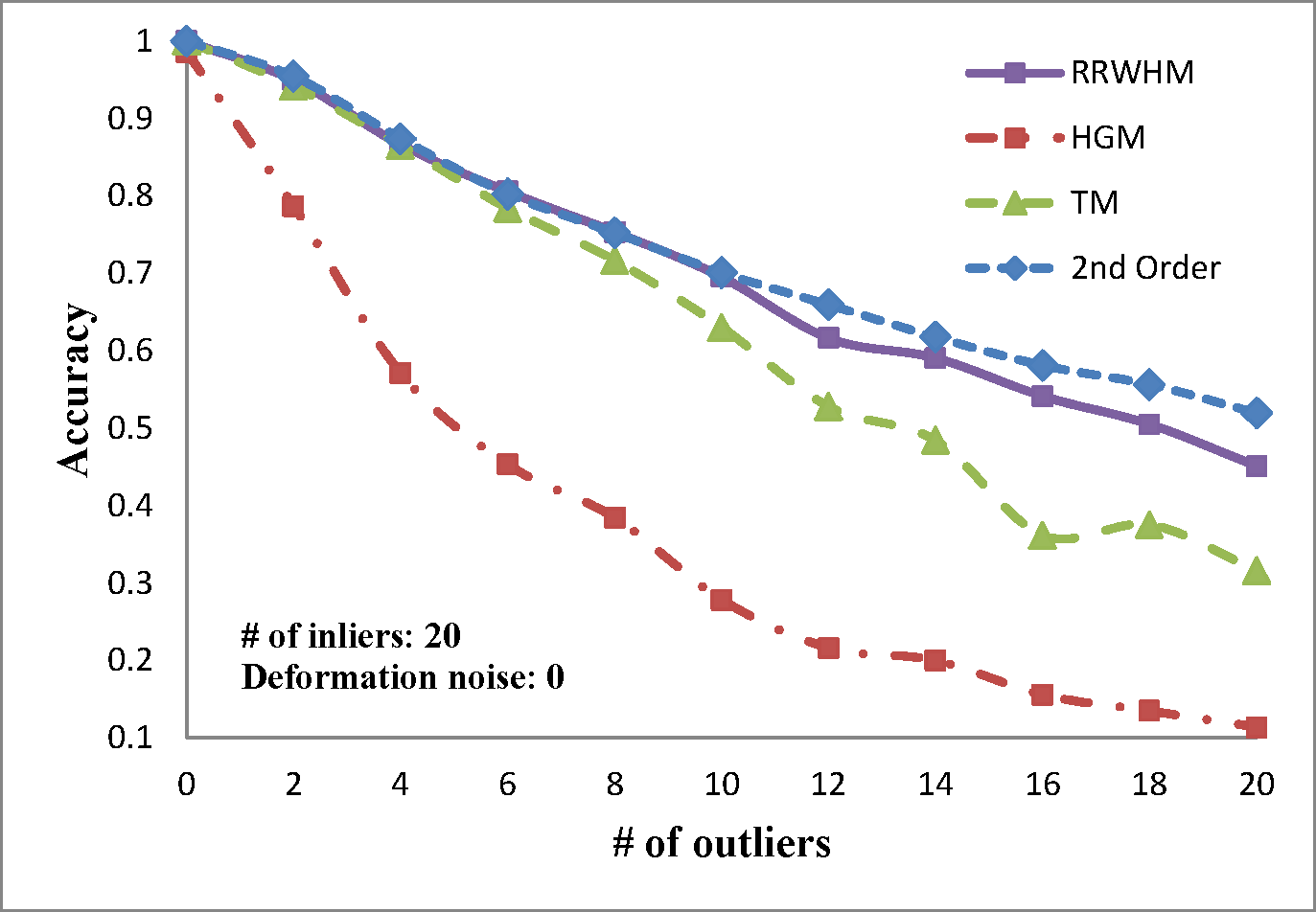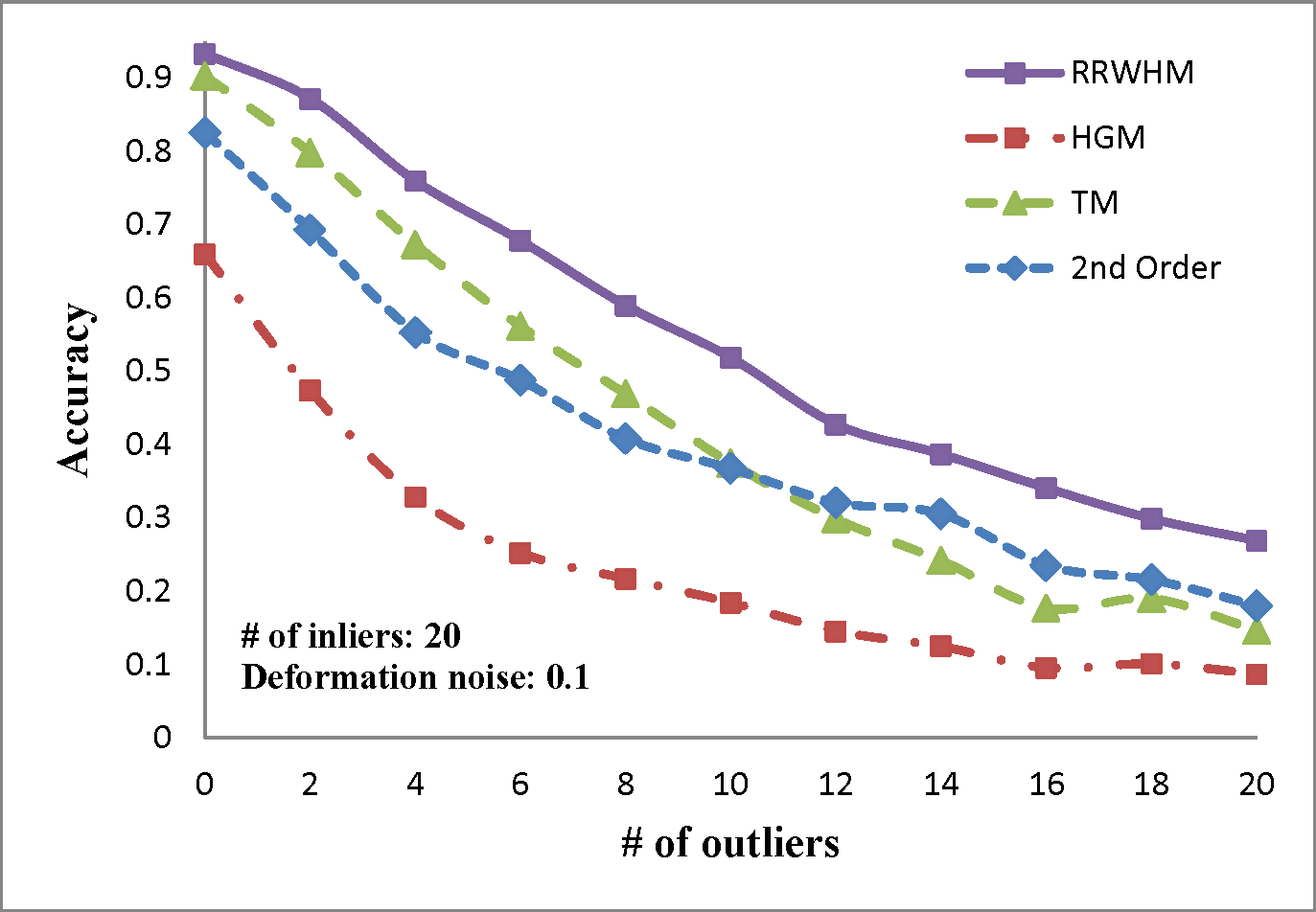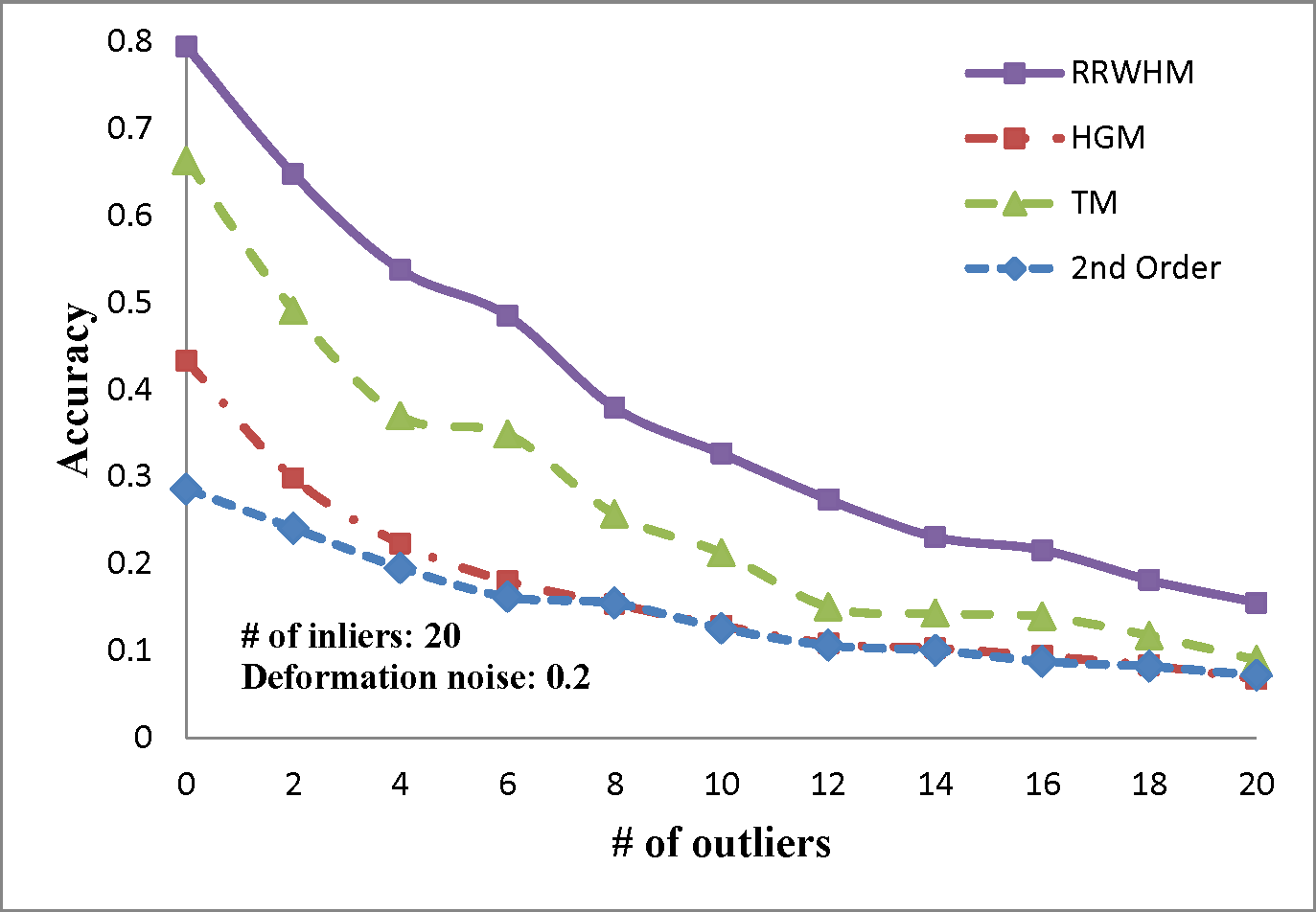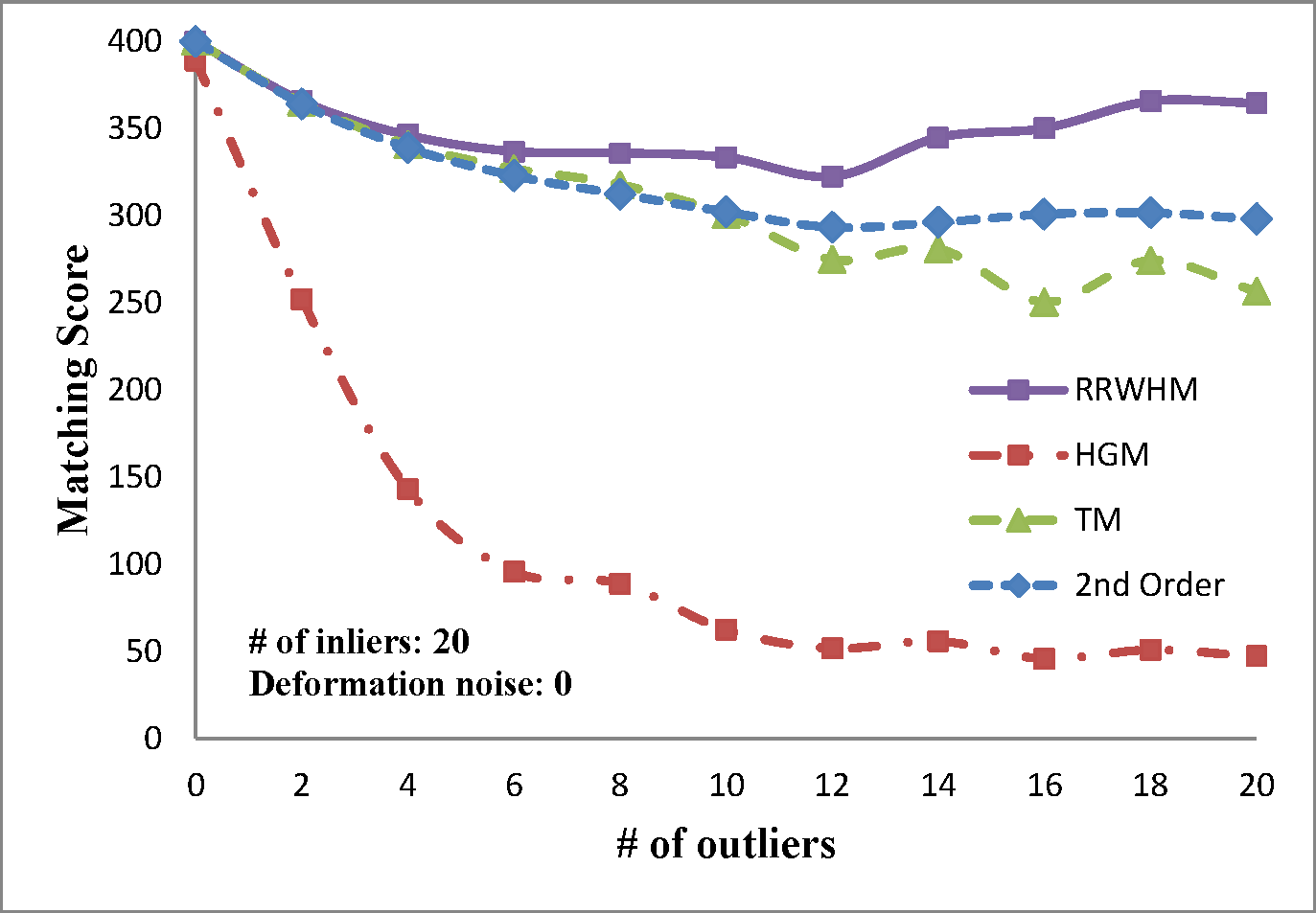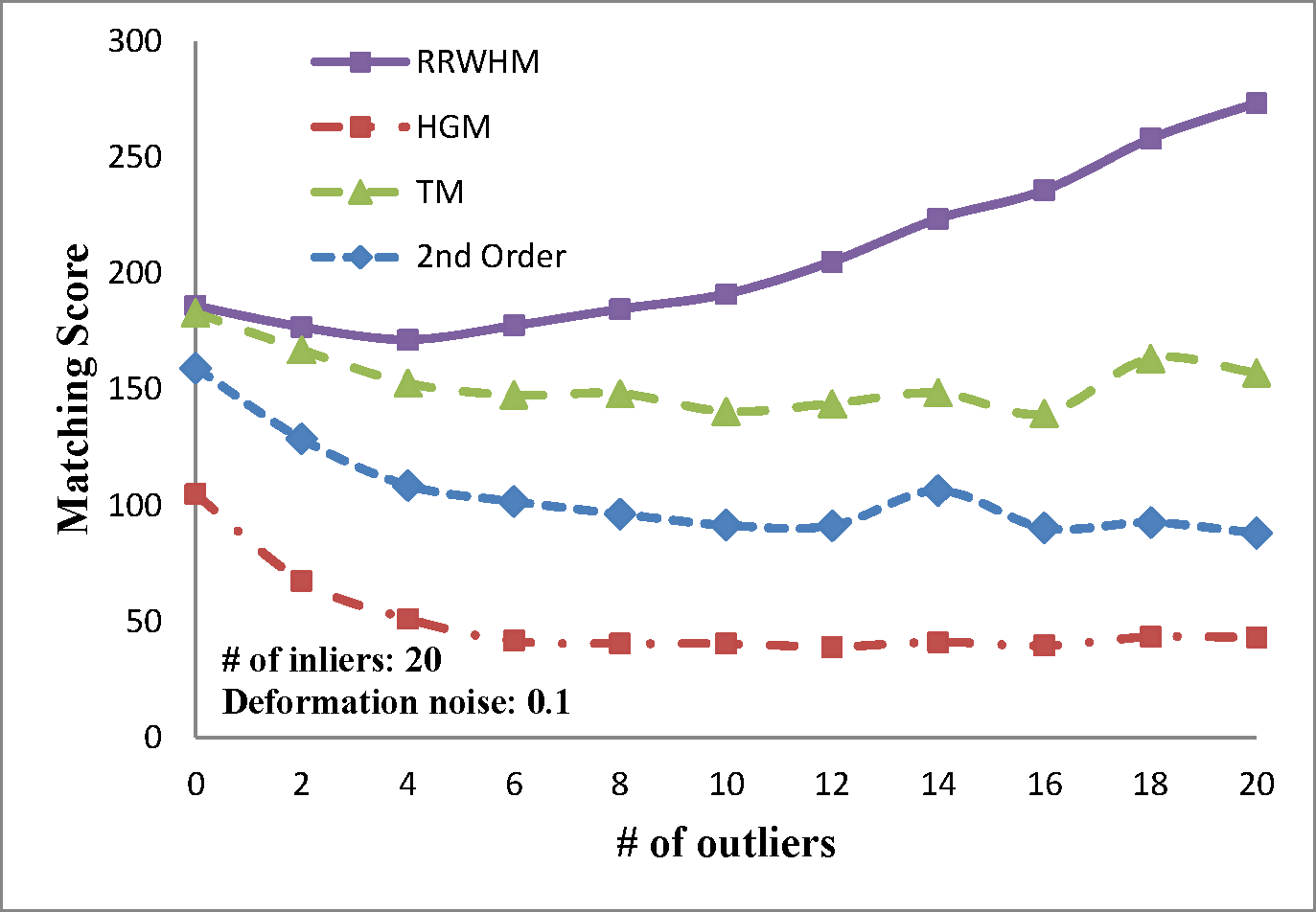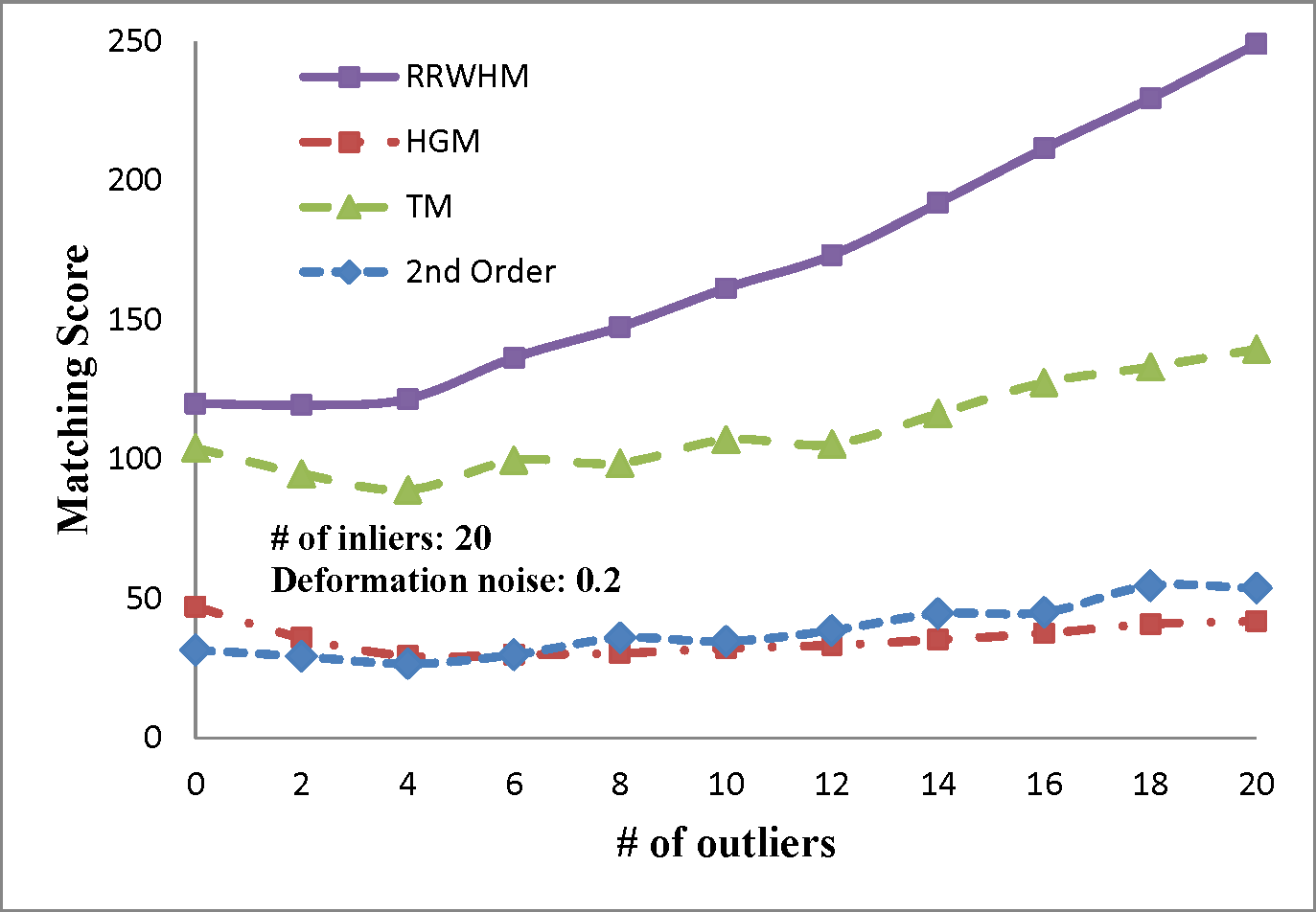The experimental results are plotted in above figures. To compare the robustness of the higher-order feature, we also evaluate the performance of the state-of-the-art pairwise graph matching algorithm denoted as '2nd Order'. In the deformation test, we vary the deformation noise σ from 0 to 0.2 with the interval 0.025 while nin is fixed to 20, 30, and 40. In the outlier noise test, the number of outliers nout varies from 0 to 20 with the interval 2, while σ is set to 0, 0.1, and 0.2.
The performances of each method are measured in both accuracy and scores. The accuracy represents the ratio between the number of correctly selected correspondences and that of the inlier points nin. The matching score is defined by S(X) in Eq. 4 of the paper. In most experiments, the proposed method outperforms the others in the sense of both the accuracy and the matching score. HGM does not gain a good performance due to the information loss at its marginalization step, and TM does not deal with the presence of outliers or large amount of deformation noise in computing the principal eigenvector of the affinity tensor. To the contrary, RRWHM clearly outperforms them since its affinity-preserving property and reweighting scheme enable to avoid adverse effects from deformation and outliers. Without deformation noise, the second-order method of  performs best in accuracy on the outlier experiment as shown at the left-top of 'Outlier Test' figure. This is mainly because pairwise distance measure has a more robust characteristic to outliers for ideal case without deformation. The second-order method, however, does not show satisfactory performance in the presence of deformation noise in all the other experiments.

1. R. Zass and A. Shashua. "Probabilistic graph and hypergraph matching", CVPR, 2008.
2. O. Duchenne, F. Bach, I. Kweon, and J. Ponce. "Tensor-based algorithm for high-order matching", CVPR, 2009.
3. M. Cho, J. Lee, and K. M. Lee. "Reweighted random walks for graph matching", ECCV, 2010.

### Image Matching Test

Image matching experimental results will be posted soon.
(We are developing a new higher-order similarity measure for better results.)